## Video Game -- Drink from the Fire Hose

Introduction.

You will produce a game in which ball-like particles enter from one side of the screen. You must catch the balls with a collector to get points. The collector position will be controlled by an analog input. There will be a time limit to the game. Display will be on an 320x240 TFT LCD, with sound effects.

The balls will follow standard billards-type dynamics, with zero friction between balls. An example of billard dynamics is shown here and slower.
The sound will be produced through the Vref output using a DMA channel.

Procedure:

In this lab and every lab, make sure you are running Protothreads 1_2_1!
There is fixed point animation example on the Protothreads page, including forces for gravity and drag. But note that the TFT display must be moved from SPI channel 1 to SPI channel 2 so that you can use the Vref output. Tahmid thoughtfully converted the TFT code to work with SPI2. Download tft_master_spi2.c and include it in the project, and, of course, remove the SPI1 master from the project. Move the TFT SCK input from pin 25 to pin 26 on the PIC32. All other TFT connections from lab 2 stay the same.
Use pins:

• TFT uses pins 4,5,6, 22 and 26 (RB0, RB1, RB2, MOSI2, SCLK2)
SCK2: connected to pin 26 on the PIC
MOSI (SDO1): PPS output group 2 connected to RB11 on the PIC
CS: connected to RB1 on the PIC
SDCS: left unconnected as I'm not using the microSD card for this
RST: connected to RB2 on the PIC
D/C: connected to RB0 on the PIC
VIN: connected to 3.3V supply
GND: connected to gnd
• Vref output pin 25. Connect the sound production Vref DAC output to speakers using the connector from lab 2.
In the Vref control register CVRCON (Reference manual chapter 20.2),
• bit 15 set turns on the Vref source
• bits 8-10 selects input reference. Choose zeros for DAC operation.
• bit 6 set connects Vref to pin 25,
• bit 5 set and bit 4 clear chooses a voltage range of` 0 to 0.67*(AVDD–AVSS)`
• bits 0-3 set one of 16 voltage levels
• Therefore to output a voltage `V` (where `V` is 4 bits) load CVRCON with `0x806V`
• ADC input AN11 pin 24 using the potentiometer circuit shown below.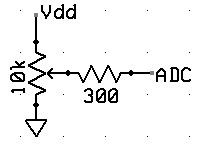The game will be controlled by a trimpot potentiometer hooked to an ADC input on the PIC32. Use the circuit to the left to
make a user-variable voltage. The Protothreads page shows how to set up the A/D converter to read a voltage in a thread.

Trimpot schematic: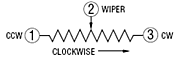bottom view: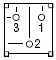image: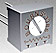An example which reads the AN11 analog input and draws the voltage on the TFT using the SPI2 master and Protothreads 1.2 is ZIPPED here.

Dynamics:

You are going to be programming in the equations of motion for the balls. Remember that the video coordinate system has x increasing to the right and y increasing downward. We will step the billards system forward in time by calculating the total change in velocity from a collision, without worrying exactly how forces change the velocity.

The change in velocity during impact can be derived for frictionless balls of equal mass by noting that the the impact force must act in a direction parallel to the line connecting the centers of the two impacting balls. The change in velocity must be parallel to the connecting line also, with the velocity component parallel to the line having its sign reversed by the collision and the velocity component perpendicular to the line unchanged. Projecting the initial velocity onto the line connecting the centers, negating the result, and resolving it back into x and y velocity components gives the velocity change. If i and j are the indices of the colliding balls, define: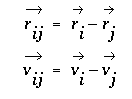then delta v for ball i is given by the following where the right-most term represents the projection of the velocity onto the line and the other term converts the projection back to x,y coordinates.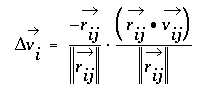The calculation procedure for each time step is:

1. Compute the Δv for each collision, based on the positions of the balls, and add it to the current velocity. Do this for every pair of balls. This step is time consuming, but only has to be performed for any two balls if they are less than 2 radii apart. Ball-ball collisions will not be exact because of finite time steps. One consequence of this is that balls tend to capture each other when they collide. You will need to do a numerical hack to avoid capture. I suggest that after a ball collides with another ball or the paddle, that it not be allowed to collide again for a few frames. For collision debugging, it will be useful to slow down particles to less than one pixel/frame.
Pseudocode for this might be:
```For each ball i from 1 to n   For each ball j from i+1 to n     Compute approximate rij by checking:     if Δx and Δy are both less than 4      if (||rij||2 less than (2*(ballRadius))2 and hitCounteri is zero)         Compute vij         Compute Δvi         Add Δvi to vi         Subtract Δvi from vj         Set hitCounteri big enough to avoid particle capture      elseif (hitCounteri>0)         decrement hitCounteri      endif     endif   endfor endfor ``` When I coded this, I did not bother to calculate the square root of the sum of squares when calculating ||`rij`|| (too slow). Compare the squares.
When dividing by ||`rij`||2 you can use the known value of `(2*ballRadius)2`. In the assignment below, I set `ballRadius=2`. This is equivalent to a shift-right by 4-bits.
2. For each ball, simulate friction between the ball and table by making
`vx(t+dt)=vx(t)-vx*drag` and ```vy(t+dt)=vy-vy*drag ```The drag should be small, perhaps` drag=0.001 `( but converted to fixed notation).
3. Update the positions according to
`x(t+dt)=x(t)+vx*dt` and` y(t+dt)=y(t)+vy*dt`
4. Detect collisions with the walls and modify velocities by negating the velocity component perprendicular to the wall.
5. Delete any balls which are collected, or which make it past the collector to the left side of the screen, and modify the score

Clearly, v and x all need initial conditions, which you will set, according the specifications below. It is doubtful that you will have enough time between frames to do all of the calculations in floating point. I suggest using 32 bit, signed numbers with the binary point set at the 16-bit boundary. I also suggest scaling velocity so that you can make dt=1, thereby avoiding a multiply. You can think of this as: (1) Units of distance are PIXELS, (2) Units of velocity are PIXELS/FRAME. As examples, any of the NTSC particle systems are done with fixed point numbers.

The previous analysis is adapted from: Studies in Molecular Dynamics. I. General Method, by B. Alder and T. Wainwright,
Journal of Chemical Physics, Vol 31 #2, Aug 1959, pp 459-466. See also Hard-Sphere molecular dynamics.
One final project in 2005 used a different scheme to calculate collisions.

You will need your digital camera to document your project.

Results:

2011: video
2014: video Shiva Rajagopal and Richard Quan
2016: video

Assignment

Write a program in C using ProtoThreads with these specifications:

• At reset, the program should:
• draw a playing field consisting of a rectangle 320 wide x 240 high on the LCD screen with two internal barriers.
The barriers should be placed at about 1/3 the x-width of the screen and and about 1/4 of the screen long.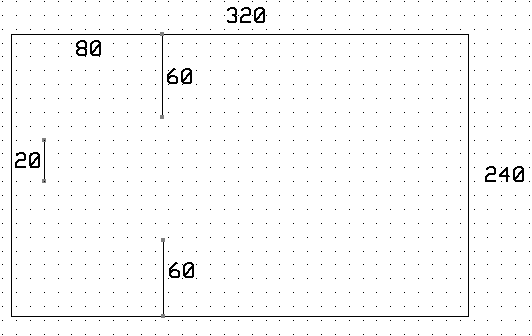• set the running time clock to zero. The clock should read elapsed play time on secreen.
• start firing balls of radius 2 pixels onto the right hand edge of the screen with vx=-1 to -3 pixels/frame and vy=+1.5 to -1.5 pixels/frame.
The speed distribution in the y-direction must be uniform and random. The x speed can be fixed between the values indicated.
You can vary this to make the game more playable, if necessary. The ball icons can be very simple.
• draw a "catcher" consisting of a line segment with a size about 5 times the size of the balls. You can vary this to make the game more playable, or to produce harder levels of play.
• Set the TFT frame time using a thread to be faster than 15/second.
Since the computation will be the most demanding calculation and depends on the number of balls,
arrange the thread to produce a constant frame rate, while allowing as much time as possible for computation.
(pseudocode example of constant frame rate)
Display the frame rate on the screen in frames/sec.
• At each frame time, update the velocity and position of all the balls on the screen, and redraw the catcher.
The drawing of the catcher need not be complicated.
• Balls which are deflected into the front of the catcher increment your score, and the balls are removed from the screen.
Balls which get past the catcher to the left side of the screen decrement your score, and the balls are removed from the screen.
• A score, a frame rate, number of current balls, and time should be displayed.
• All balls can be deflected by other balls according to the hard ball dynamics given above.
• Catcher vertical position on the screen should be changed by a potientiometer attached to the A/D converter.
The catcher horizontal position will be fixed at around x=20.
• The game ends after a fixed time, which you can choose.
• New balls should enter the playing field at regular intervals (from the right hand edge), perhaps a few per second. The oldest ball will be removed from the screen.
• You will be graded on the number of simultaneous balls you can animate. You must animate at least 30 balls.
You will need to optmize your code and use fixed point.
• There should be minimal visual artifacts (tearing, flickering) during operation.
• There are required sound effects, which for full credit, must be generated using DMA-driven, Vref DAC output.
You need a sound for +1 score, -1 score, and for game end.
Backgound music optional.

When you demonstrate the program to a staff member, you should play the game.
At no time during the demo should you need to press RESET.

Your written lab report should include the sections mentioned in the policy page, and :

• The DMA setup for Vref output..
• The details of your integration algorithm.
• An image from the TFT LCD (bring your camera).
• A heavily commented listing of your code.

Copyright Cornell University
October 14, 2016
sent with 100% recycled electrons.